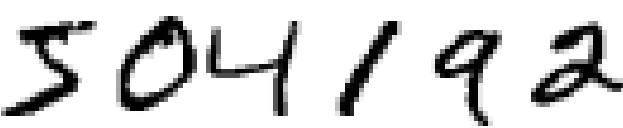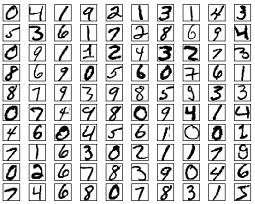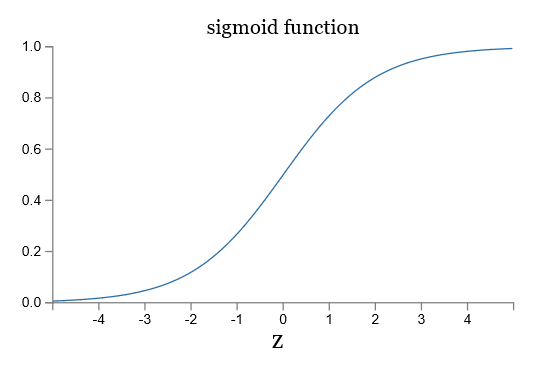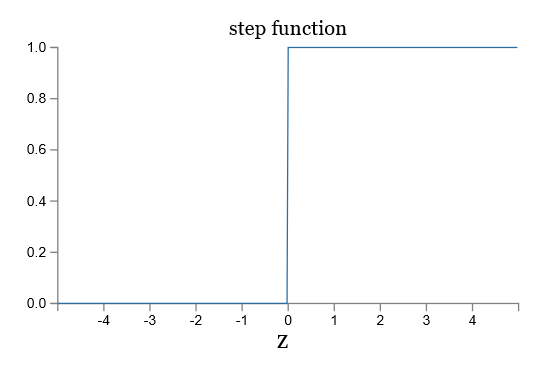# 基于 BP 神经网络的识别手写体数字 - 神经网络基础 (基于 BP 神经网络的识别手写体数字, Part 1)

## 前言## 感知器 Perceptron\begin{equation}
output = \left\{\begin{aligned} 0, \sum_jw_jx_j \leq threshold \\
1, \sum_jw_jx_j > threshold \end{aligned}\right. \end{equation}

1. 天气怎样？
2. 你的女朋友和你一起去吗？
3. 节日举办地驾车方便吗？\begin{equation}
output = \left\{\begin{aligned} 0, w \cdot x + b \leq 0 \\
1, w \cdot x + b > 0 \end{aligned}\right. \end{equation}

## sigmoid 神经元\begin{equation}
\sigma(z) \equiv \frac{1}{1+e^{-z}}. \end{equation}

\begin{equation} \frac{1}{1+\exp(-\sum_j w_j x_j-b)}. \label{eq4} \end{equation}\begin{eqnarray} \Delta \mbox{output} \approx \sum_j \frac{\partial , \mbox{output}}{\partial w_j} \Delta w_j + \frac{\partial , \mbox{output}}{\partial b} \Delta b, \label{5} \end{eqnarray}

## 神经网络的结构